Performing narrow-band magnetic-field spectroscopy with NV centers

Using Boulder Opal spectrum reconstruction tools to perform provably optimal leakage-free sensing with spectrally concentrated Slepian pulses

Boulder Opal provides flexible tools for noise characterization and spectroscopy which can be applied to state-of-the art quantum sensors. One key quantum sensor for magnetic fields operates based on Nitrogen-Vacancy (NV) centers in diamond. The NV center is a spin-1 defect in diamond of significant experimental interest to room temperature quantum magnetometry and nano nuclear magnetic resonance (nano-NMR) spectroscopy, as well as sensing of electric fields, temperature and pressure in exotic environments. In practice, spectroscopy with NV centers typically relies on dynamic-decoupling-pulse sequence-based power spectrum reconstruction methods, such as the Suter–Álvarez (SA) method. Unfortunately, these pulse-based techniques can suffer out-of-band spectral leakage that can lead to signal misidentification.

In this application note, we demonstrate a flexible protocol allowing you to use special controls which suppress spectral leakage. We will cover:

• Analyzing spectral leakage in dynamic decoupling using filter functions
• Designing provably optimal probe controls which suppress spectral leakage
• Using machine learning tools to efficiently perform spectrum reconstruction with any set of controls

We will demonstrate an ability to simply perform magnetic field spectroscopy using NV centers and state-of-the-art machine learning protocols to improve flexibility in measurements, reduce out-of-band sensitivity to background interference to the lowest levels allowed by mathematics, and improve the accuracy of signal reconstruction via minimization of leakage-induced artifacts.

A detailed comparison of Slepian vs SA reconstruction appears in Frey et al published as Phys. Rev. Applied 14, 024021 (2020).

Imports and initialization

import jsonpickle
import matplotlib.pyplot as plt
import numpy as np
from qctrlvisualizer import get_qctrl_style

plt.style.use(get_qctrl_style())

from qctrl import Qctrl

# Starting a session with the API
qctrl = Qctrl()

# Helper functions
def save_var(file_name, var):
# Save a single variable to a file using jsonpickle
f = open(file_name, "w+")
to_write = jsonpickle.encode(var)
f.write(to_write)
f.close()

# Return a variable from a json file
f = open(file_name, "r+")
decoded = jsonpickle.decode(encoded)
f.close()
return decoded

def first_order_slepian_based_controls(
maximum_rabi_rate, duration, number_of_segments, frequency
):
"""
This function provides spectrally concentrated controls derived from first-order Slepian functions.
"""
times = np.arange(0, number_of_segments + 1, 1) * duration / (number_of_segments)
slepian_envelope = np.kaiser(number_of_segments + 1, 2 * np.pi)
total_power = np.sum(np.abs(slepian_envelope * number_of_segments) ** 2)

# COS shift
cos_modulation = np.cos(2 * np.pi * frequency * times)
slepian = cos_modulation * slepian_envelope

# Compute the time derivative to obtain dephasing sensitivity
slepian = slepian[:-1] - slepian[1:]

# Rescale to maintain power
slepian = slepian * np.sqrt(
total_power / np.sum(np.abs(slepian * number_of_segments) ** 2)
)

# Return control as dict
segment_duration = duration / (number_of_segments)
phasors = maximum_rabi_rate * slepian + 0 * 1j
return {
"operator": sigma_m / 2.0,
"control": [(segment_duration, v) for v in phasors],
}

sigma_z = np.array([[1.0, 0.0], [0.0, -1.0]], dtype=complex)
sigma_x = np.array([[0.0, 1.0], [1.0, 0.0]], dtype=complex)
sigma_y = np.array([[0.0, -1.0j], [1.0j, 0.0]], dtype=complex)
sigma_m = np.array([[0.0, 1.0], [0.0, 0.0]], dtype=complex)

data_folder = "./resources/nitrogen-vacancy-centers-narrow-band-spectroscopy/"

Model of the NV center and the magnetic spectrum

In this notebook, we use a single NV center to interrogate a given magnetic field spectrum over a specific window. We begin with a brief look at the relevant NV-center Hamiltonian.

For the purposes of general magnetic field sensing, the ground state of the negatively charged NV center is well described by the following Hamiltonian:

\begin{align*} H = DS_z^2 + \gamma_e B_0 S_z + \gamma_eB_1(\cos(\omega t +\phi)S_x + \sin(\omega t +\phi)S_y) + \gamma_e B_{AC} S_z, \end{align*}

where $S_{x,y,z}$ are the $S=1$ spin operators and $\gamma_e$ is the gyromagnetic ratio of an electron. The $|\pm1\rangle$ levels are separated from $|0\rangle$ by the zero-field splitting $D=2.87{\rm GHz}$. The background magnetic field $B_0$ is applied along the direction of the NV center quantization axis. The driving field used to control the spin transitions has an amplitude $B_1$, frequency $\omega$, and relative phase $\phi$. Finally, $B_{AC}$ represents the component of the magnetic spectrum fluctuations in the direction of the NV center axis.

In sensing applications, it's typical to run pulse sequences on one of two spin transitions. Setting $\omega=D-\gamma_eB_0$ allows for resonant control of the $|0\rangle \leftrightarrow |-1\rangle$ transition without populating the $|+1\rangle$ level under usual experiment conditions ($D+\gamma_eB_0\gg \gamma_eB_1$). Therefore, this system can be effectively reduced to the following two level Hamiltonian:

\begin{align*} H = D\frac{\sigma_z}{2} - \gamma_e B_0 \frac{\sigma_z}{2} + \gamma_eB_1\left(\cos(\omega t +\phi)\frac{\sigma_x}{2} + \sin(\omega t +\phi)\frac{\sigma_y}{2}\right) + \gamma_e B_{AC} \frac{\sigma_z}{2}, \end{align*}

where $\sigma_{x,y,z}$ are Pauli matrices. Finally, moving into the rotating frame, the Hamiltonian can be expressed as:

\begin{align*} H = \Omega\frac{\sigma_x}{2} + \gamma_e B_{AC} \frac{\sigma_z}{2}, \end{align*}

where the driving field has been absorbed into the complex Rabi rate $\Omega= \gamma_e B_1e^{i\phi}$.

We explore the relevance of the control protocol and spectral reconstruction algorithm in reconstructing a synthesized magnetic field spectrum from sensor measurements. The magnetic spectrum we examine is shown below, representing an arbitrary complex environmental landscape containing multiple features - a trait found in various scenarios, from sensing macroscopic objects to nano-NMR.

We interrogate a specific window of the spectrum from $\nu_{\rm min}$ to $\nu_{\rm max}$, here taken to be in the low MHz range. The particular range of the window (e.g., whether MHz, kHz, etc.) is arbitrary; however, as we show later, it's essential to note that the spectrum contains features outside of the interrogation window.

spectrum_frequencies = spectrum_data["spectrum_frequencies"]
spectrum = spectrum_data["spectrum"]

# Interrogation window
lower_sample_frequency = 2e4
upper_sample_frequency = 2e6
sample_selection = (spectrum_frequencies >= lower_sample_frequency) & (
spectrum_frequencies <= upper_sample_frequency
)
sample_frequencies = spectrum_frequencies[sample_selection]
sample_spectrum = spectrum[sample_selection]

# Number of sampling points over the window
number_of_sample_points = 50

# Plot
fig, ax = plt.subplots(figsize=(15, 5))
ax.plot(spectrum_frequencies / 1e6, spectrum / 1e3, label="Full spectrum", color="gray")
ax.fill_between(spectrum_frequencies / 1e6, spectrum / 1e3, 0, color="lightgray")

ax.plot(sample_frequencies / 1e6, sample_spectrum / 1e3, label="Interrogation window")

ax.set_xlabel("Frequency (MHz)")
ax.set_ylabel("Power density (kWHz$^{-1}$)")
ax.legend()
fig.suptitle("Magnetic field power spectrum")
plt.show()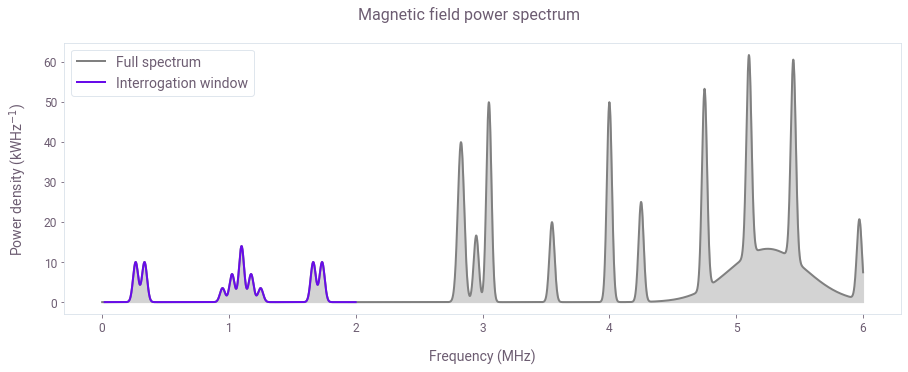The window we interrogate is highlighted (purple), while the spectrum contains other features outside of it (gray).

Spectrum reconstruction with Suter–Álvarez

Conventional spectroscopy with NV centers often relies on pulse sequences of Carr-Purcell-Meiboom-Gill (CPMG) or XY families, whose filter functions have nontrivial profiles. They are characterized by higher frequency harmonics which interfere with spectrum reconstruction. This issue leads to the appearance of spectrum-dependent artifacts and errors in peak magnitudes.

In this section, we use the SA method to illustrates how out-of-band leakage causes issues with conventional approaches to spectrum reconstruction. The SA technique uses a series of CPMG sequences of fixed duration. It accounts for their harmonics by carefully choosing the sequence order to ensure overlaps between the fundamental and harmonic frequencies of successive sequences. This can be effective in partially mitigating the effects of harmonics in the filter functions, but cannot do so completely and simultaneously adds strict requirements on which measurement sequences may be used.

Filter functions of sequences in conventional spectrum reconstruction approaches

Below we calculate and display the filter functions of all the CPMG sequences used to probe the spectral window of interest. We observe multiple harmonics located outside the interrogation window, a common feature of conventional dynamic decoupling protocols.

# Max Rabi rate of pulses
maximum_rabi_rate = 2.0 * np.pi * 20 * 1e6

# Duration of CPMG sequences
duration = 100e-6

# Shot noise
shot_noise = 1e-4

SA_frequencies = SA_data["frequencies"]
SA_filter_functions = SA_data["filter_functions"]

# Plot
fig, ax = plt.subplots(figsize=(15, 5))
for ff in SA_filter_functions:
ax.plot(SA_frequencies / 1e6, ff, linewidth=0.8)

fig.suptitle("CPMG filter functions/Power spectrum")
ax.set_xlabel("Frequency (MHz)")
ax.set_ylabel("Inverse power (W$^{-1}$)")
ax2 = ax.twinx()
ax2.set_ylabel("Spectrum power")

ax2.fill_between(spectrum_frequencies / 1e6, spectrum / 1e3, 0, alpha=0.2, color="k")

plt.show()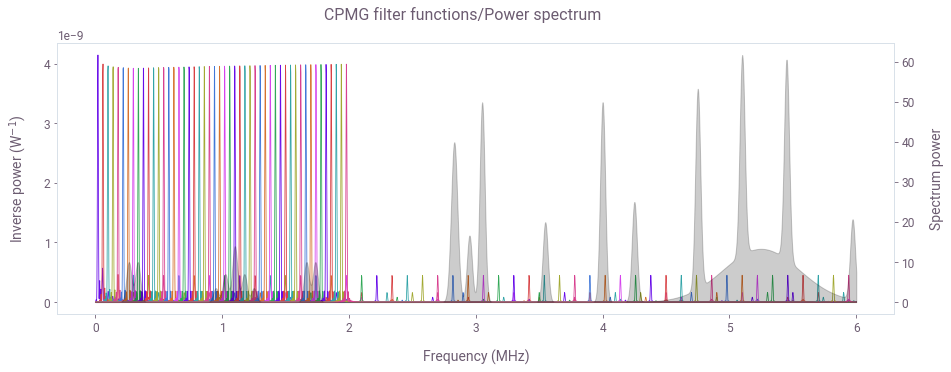Shown above are the filter functions of CPMG sequences spanning the interrogation window under the SA arrangement. Note that the higher order harmonics cover a significant portion of the spectrum outside the interrogation window. Those harmonics pick up the contributions from the unaccounted spectral components creating errors and artifacts in the reconstruction of the sampled spectrum.

Measurements

The measurements for each of the CPMG sequences would be collected experimentally, however, here they have been simulated using the filter function framework. The probability of finding the NV center in the $m=-1$ state can be estimated as $P_i= \int F^i(\nu)S(\nu)d\nu + \epsilon_m$, where the $F^i(\nu)$ is the filter function of the $i^{th}$ CPMG sequence and $S(\nu)$ is the magnetic power spectrum density. For clarity, the shot noise $\epsilon_m$ is set to be negligible (standard deviation $\sigma=10^{-4}$).

SA_measurements = [m["measurement"] for m in SA_data["measurement_list"]]

fig, ax = plt.subplots(figsize=(15, 5))
ax.plot(SA_measurements, label="", color="k")
ax.set_xlabel("Sequence index")
ax.set_ylabel("Probability")
fig.suptitle("SA measurements")
plt.show()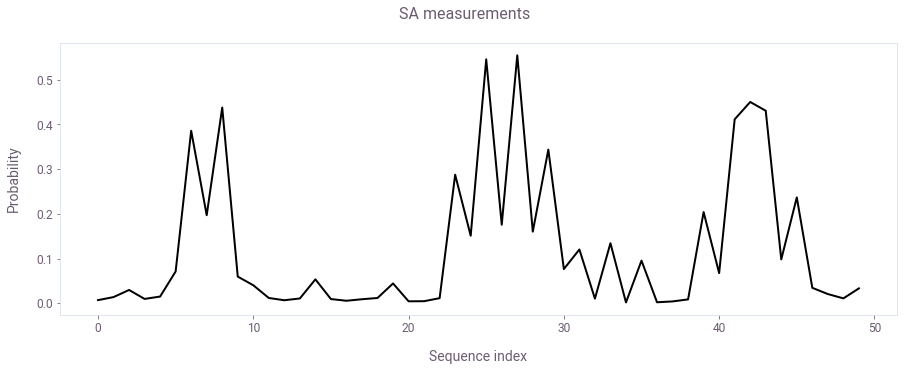Probability of the $m=-1$ NV state for the index of the CPMG sequence.

Suter–Álvarez reconstruction

The SA algorithm takes in the measurements associated with the CPMG sequences and produces the power spectrum shown below using the method developed by G. A. Álvarez and D. Suter.

SA_reconstructed_frequencies = SA_data["reconstructed_frequencies"]
SA_reconstructed_power = SA_data["reconstructed_power"]

fig, ax = plt.subplots(figsize=(15, 5))
ax.plot(sample_frequencies / 1e6, sample_spectrum / 1e3, color="gray")
ax.fill_between(
sample_frequencies / 1e6,
sample_spectrum / 1e3,
0,
color="lightgray",
label="True spectrum",
)
ax.plot(
SA_reconstructed_frequencies / 1e6,
SA_reconstructed_power / 1e3,
"-",
color="#FF3860",
label="SA reconstructed spectrum",
)
ax.set_xlabel("Frequency (Mhz)")
ax.set_ylabel("Power density (kWHz$^{-1}$)")
ax.legend()

fig.suptitle("Suter–Álvarez power spectrum reconstruction")
plt.show()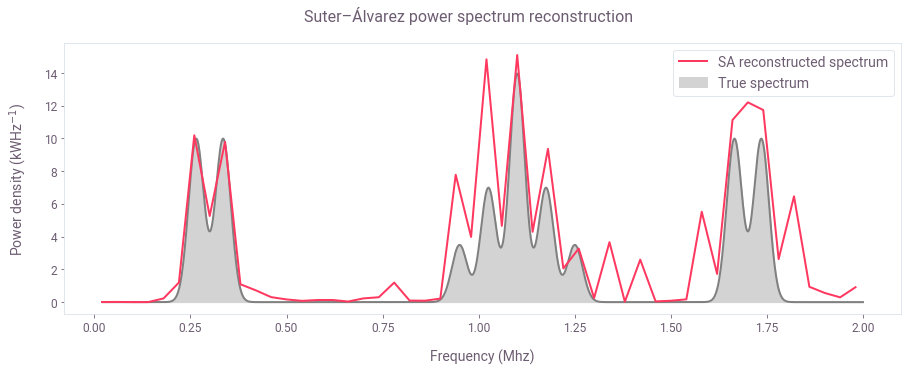The interrogated section of the spectrum highlights discrepancies between the true spectrum (gray) and the spectrum reconstructed with the Suter–Álvarez technique (red). We observe that in comparison to the plot of measurements in the section above, the SA technique managed to correct the artifacts caused by harmonic within the interrogation window as seen around the feature on the left. However, SA misses some of the features of the real spectrum while simultaneously adding spurious ones due to the effects of out-of-band harmonic leakage, as seen in features in the middle and right. In this example, the amount of power that has leaked into the interrogation window is around $67\%$.

Spectrum reconstruction with Boulder Opal

We perform spectrum reconstruction using an alternate method based on narrowband Slepian functions. An overview of the general method is available the Boulder Opal noise characterization user guide.

Slepian-based controls

Here we use first order Slepians to derive spectrally concentrated pulses that probe specific frequencies free of higher-order harmonics. These pulses will have the same duration as the CPMG sequences above, however, they'll be composed of the time derivative of the continuous Slepian waveform modulated by the frequencies you want to interrogate in order to ensure sensitivity in the $\sigma_{z}$ quadrature associated with sensing of a magnetic field.

Below is the array of Slepian-based controls; each constitutes a variable width envelope combined with a modulation whcih shifts the band-center of the control's response.

# Points at which to probe the frequency window
number_of_sample_points = 100
slepian_frequencies = np.linspace(
lower_sample_frequency, upper_sample_frequency, number_of_sample_points
)

# Set up gradually varying Rabi rates to make filter function amplitudes constant
slepian_rabi_rate_0 = 2 * np.pi * 15e3
slepian_rabi_rates = slepian_rabi_rate_0 * slepian_frequencies / slepian_frequencies

# Number of segments the Slepian pulse is discretized into
number_of_segments = 4000

# Generate the array of Slepian-based controls
slepian_based_controls = [
first_order_slepian_based_controls(
rabi_rate, duration, number_of_segments, frequency
)
for frequency, rabi_rate in zip(slepian_frequencies, slepian_rabi_rates)
]
# Plot the Slepian-based controls
slepian_rabi_rates_for_plot = np.array(
[
[np.real(value) for _, value in control["control"]]
for control in slepian_based_controls
]
)

slepian_durations_for_plot = [
[duration for duration, _ in control["control"]]
for control in slepian_based_controls
]

slepian_times_for_plot = [
np.cumsum(durations) for durations in slepian_durations_for_plot
]

for idx in [10, 20, 80]:
fig, ax = plt.subplots(figsize=(14, 3))
ax.plot(
slepian_times_for_plot[idx] / 1e-6,
slepian_rabi_rates_for_plot[idx] / np.pi / 2 / 1e6,
)

ax.set_ylabel("Rabi rate (MHz)")
ax.set_xlabel("Time ($\mu s$)")
fig.suptitle(
"Slepian-based pulse for sampling frequency: "
f"{slepian_frequencies[idx] / 1e6:.2f} MHz"
)
plt.show()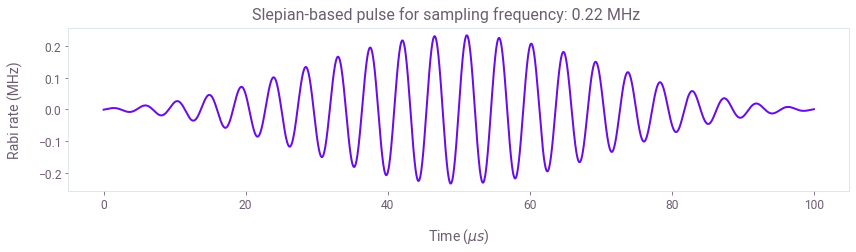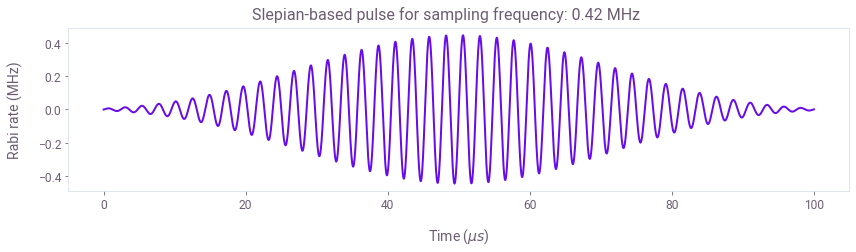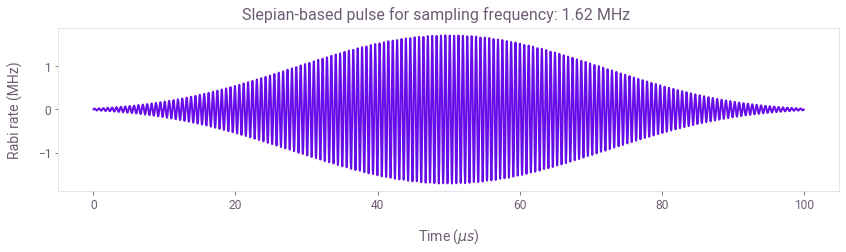Above, we present a selection of the Slepian-based pulses. The frequency that each control is set to probe is controlled by modulation of the Slepian envelope. An advantage of these controls is that you can keep them at a fixed duration while continuously sweeping across the spectrum.

Next we compute the filter functions of the Slepian-based bulses. There is an option to use pre-computed results for faster evaluation.

use_saved_data = True

if use_saved_data:
else:
filter_function_data = [
qctrl.functions.calculate_filter_function(
duration=duration,
frequencies=spectrum_frequencies,
sample_count=2000,
drives=[
qctrl.types.filter_function.Drive(
control=[
qctrl.types.ComplexSegmentInput(duration=d, value=v)
for d, v in control["control"]
],
operator=control["operator"],
)
],
drifts=[
qctrl.types.filter_function.Drift(operator=sigma_z / 2, noise=True)
],
)
for control in slepian_based_controles
]

# Collect the control-frame operators in frequency domain
slepian_frequency_domain_control_operators = [
np.asarray([sample.frequency_domain_noise_operator for sample in ffd.samples])
for ffd in filter_function_data
]

# Take the y-axis projection in the control frame
# as the y component contributes to the measurements
cf_axis = sigma_y
slepian_frequency_domain_control_operators_y_proj = [
[np.trace(np.matmul(op, cf_axis)) * cf_axis for op in nops]
for nops in slepian_frequency_domain_control_operators
]

# Compute the filter functions in the subspace
slepian_filter_functions = [
np.sum(np.abs(nops_y) ** 2 / 2, axis=(1, 2))
for nops_y in slepian_frequency_domain_control_operators_y_proj
]

# Save results
save_var(data_folder + "slepian_filter_functions", slepian_filter_functions)
# Plot
fig, ax = plt.subplots(figsize=(15, 5))
for ff in slepian_filter_functions:
ax.plot(spectrum_frequencies / 1e6, ff, linewidth=0.8)

ax.set_xlabel("Frequency (MHz)")
ax.set_ylabel("Inverse power (W$^{-1}$)")
fig.suptitle("Slepian filter functions/Power spectrum")

ax2 = ax.twinx()
ax2.fill_between(spectrum_frequencies / 1e6, spectrum / 1e3, 0, alpha=0.25, color="k")
ax2.set_ylabel("Spectrum power")
plt.show()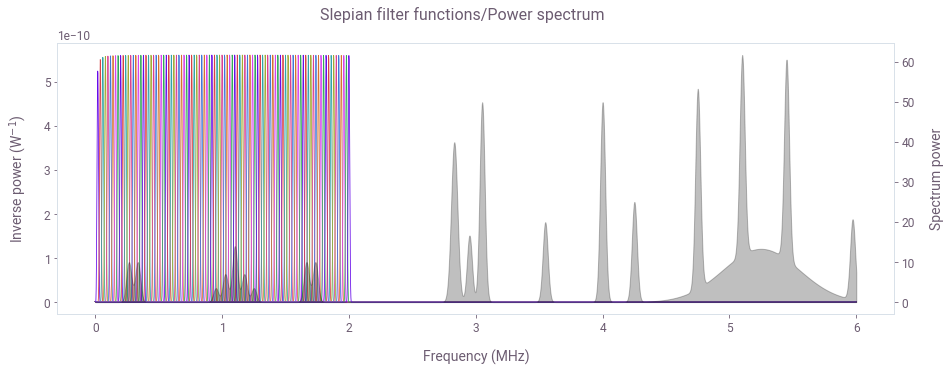As shown above, the Slepian-based pulses have a highly concentrated frequency response enabling interrogation of an arbitrary section of the spectrum without out-of-band leakage. The reconstruction procedure we use here can accommodate various sampling strategies - there are no strict requirements on the form of the sequences used as the reconstruction algorithm in Boulder Opal is much more flexible than the original SA technique. With the selected controls and their respective bandwidths we form an array of overlapping samples in a manner that ensures high-resolution sampling of the interrogation window.

Measurements

The measurements for Slepian-based controls are calculated using the filter function framework as described before; in experimental applications these would be derived directly from measurements on hardware.

# Simulated measurements
shot_noise = 1e-4

slepian_measurements = []
for ff in slepian_filter_functions:
measurement = np.trapz(ff * spectrum, x=spectrum_frequencies)
# Shot noise
measurement += np.random.default_rng().normal(0.0, shot_noise, 1)
slepian_measurements.append(np.abs(measurement))

# Plot
fig, ax = plt.subplots(figsize=(15, 5))

ax.plot(slepian_frequencies / 1e6, slepian_measurements, color="k")
ax.set_xlabel("Frequency (MHz)")
ax.set_ylabel(r"Probability")
fig.suptitle("Slepian measurements")
plt.show()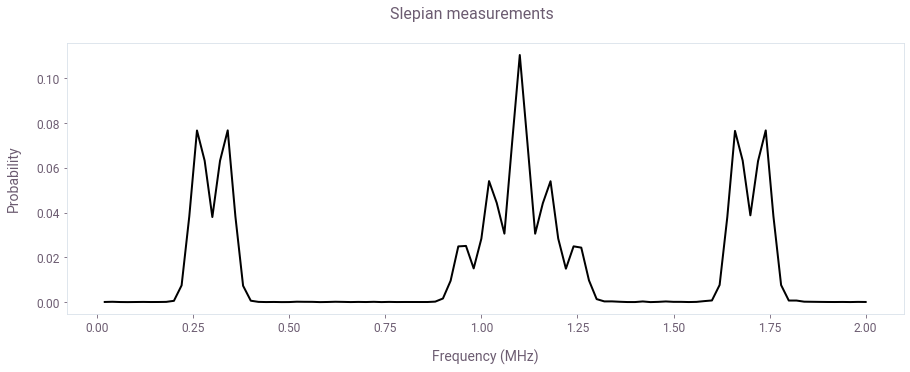The raw measurements obtained using the Slepian-based controls are free of artifacts and already reflect the spectrum closely.

Reconstruct the power spectrum with high accuracy

To capture the full frequency response of your controls, the mapping $\mathbf{M}$ between the measurements $\mathbf{P}$ and the power spectrum $\mathbf{S}$ will be based on the filter function framework. The measurement associated with the $i^{th}$ control pulse can be expressed in discretized form as follows: \begin{align*} P_i = \sum_{j=1}^N F^i_j S_j \Delta \nu, \end{align*} where the filter function of the control $F^i$ and the spectrum $\mathbf{S}$ are sampled with spectral resolution $\Delta \nu = (\nu_{\rm max}-\nu_{\rm min})/N$. Therefore, scaling the filter functions of the selected controls by $\Delta \nu$ and arranging them in a matrix enables construction of $\mathbf{M}$, such that $\mathbf{P} = \mathbf{M}\mathbf{S}$.

In the final step, we invert the mapping $M$ in order to reconstruct the interrogated section of the spectrum $\mathbf{S} = \mathbf{M^{-1}}\mathbf{P}$. Boulder Opal offers two different efficient inversion procedures powered by TensorFlow, and purpose built with spectral reconstruction in mind. They are based on single value decomposition (SVD) and convex optimization (CVX).

Both methods provide parameter free estimation needed for reconstruction of unknown power spectrum densities. The SVD procedure offers high numerical efficiency for smooth, slow-varying spectra, but may introduce unphysical oscillations in the reconstructed spectrum when these conditions are not met. By enforcing strict positivity, the CVX inversion procedure provides superior results for spectra exhibiting abrupt changes and rapid variations, as in the current example. For this reason, we use the CVX procedure in the reconstruction implementation below.

For more details and a comparison between the reconstruction methods, refer to Ball et al., as well as our noise spectrum reconstruction user guide.

# First build the measurement records with the filter function and infidelities generated above
measurement_records = []
sample_filter_functions = np.asarray(slepian_filter_functions)[:, sample_selection]

for idx, filter_function in enumerate(sample_filter_functions):
filter_function_samples = [
qctrl.types.noise_reconstruction.FilterFunctionSample(
frequency=frequency, inverse_power=inverse_power
)
for frequency, inverse_power in zip(sample_frequencies, filter_function)
]
measurement_record = qctrl.types.noise_reconstruction.Measurement(
infidelity=slepian_measurements[idx],
filter_functions=[
qctrl.types.noise_reconstruction.FilterFunction(
samples=filter_function_samples
)
],
)
measurement_records.append(measurement_record)
# Configure CVX reconstruction method
cvx_configuration = qctrl.types.noise_reconstruction.ConvexOptimization(
power_density_lower_bound=0,
power_density_upper_bound=1e9,
regularization_hyperparameter=1e-12,
)

# Perform reconstruction with CVX
noise_reconstruction_result_cvx = qctrl.functions.calculate_noise_reconstruction(
measurements=measurement_records,
method=qctrl.types.noise_reconstruction.Method(
convex_optimization=cvx_configuration
),
)

# Extract reconstructed PSD
psd_samples = noise_reconstruction_result_cvx.power_spectral_densities.samples
reconstructed_psd = np.array([sample.power_density for sample in psd_samples])
Your task calculate_noise_reconstruction (action_id="625299") has started. You can use the qctrl.get_result method to retrieve previous results.

# Plot
fig, ax = plt.subplots(figsize=(15, 5))
ax.fill_between(
sample_frequencies / 1e6,
sample_spectrum / 1e3,
0,
color="lightgray",
label="True spectrum",
)
ax.plot(
sample_frequencies / 1e6,
reconstructed_psd / 1e3,
"-",
label="Boulder Opal reconstructed spectrum",
)
ax.set_xlabel("Frequency")
ax.set_ylabel("Power density (kWHz$^{-1}$)")
ax.legend()
fig.suptitle("Power spectrum reconstruction with Boulder Opal")
plt.show()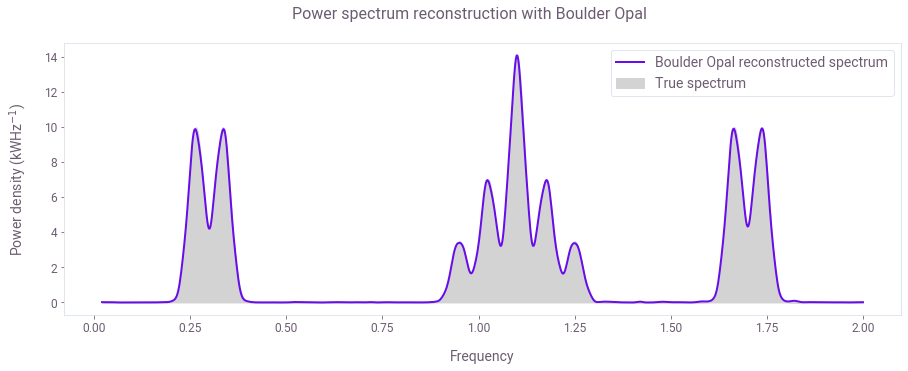By basing the reconstruction on detailed spectral response of your controls, Boulder Opal provides full flexibility in designing customized controls and sampling strategies to probe any noise spectrum. The plot above shows a highly accurate power spectrum reconstruction using Boulder Opal tools for the case where concentrated, harmonic-free Slepian controls are used.